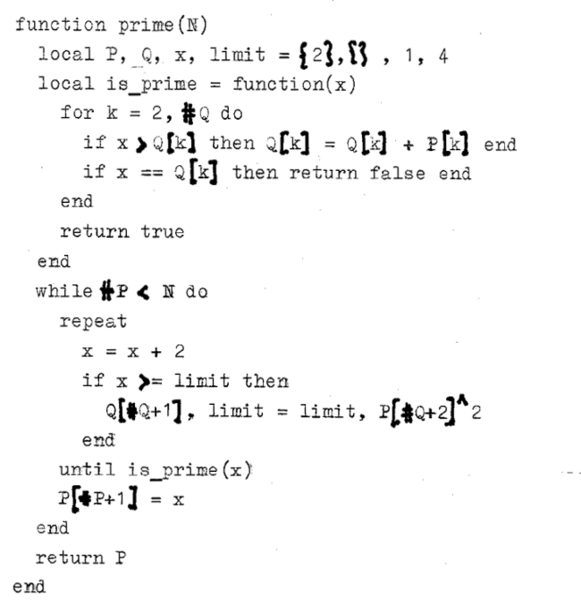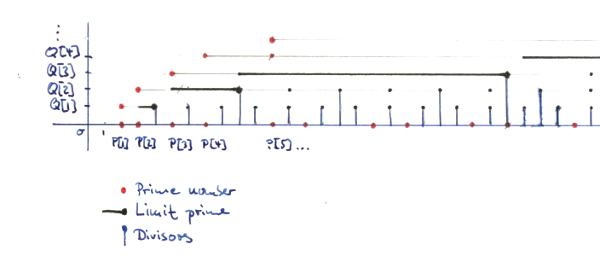Heinrich Hartmann

# Dijkstra's Prime Number Algorithm

Written on 2016-04-03

I was reading Tanenbaum’s paper  lately, which contains an algorithm for calculating prime numbers attributed to E.W. Dijkstra . What is remarkable about this algorithm is, that it uses no divisions at all! 1 Just a very innocent looking check for inequality is performed to single out divisible numbers.

The algorithm was given as an example for a special purpose language SAL. Here is a literal translation of this algorithm to lua, in its full glory:

By running this program, you can quickly verify that it produces a a list of the first 100 prime numbers. (The 100th prime number is 541, who would have thought?). But even after looking at the algorithm for a while, I was not quite able to make sense out of it. Can you?

## A refactored version

Let’s see if we can make this rather acrane program more readable by refactoring it into a form that is more ‘modern’ idiomatic and easier to comprehend. The result is the following listing:Figure 1: A refactored version of Dijkstras prime number algorithm [PNG]

Aside: As you can see, this algorithm also served as the perfect material for testing out my shiny old “Triumph Durabel” typewriter, from the 1940ies.

If you don’t like typewriters, you can have a look a the code on Github. The commit history, shows how you can arrive at this refactored version in 14 simple transformations, that did not change the results of the computation, such as:

• Change variable names.
• Don’t use print statements for output, but return a table.
• Remove iteration indices I and K by using the #-operator, to get the table size.
• Introduce the a function is_prime that calculates and returns the PRIM flag.

While making these changes the logic of the calculation became more apparent to me. I hope that others might find this version also easier to read.

## How does it work?

So, what goes into the workings of this algorithm?

The table P contains the list of already computed prime numbers.

The nuber x is the current active prime candidate, which runs over the odd numbers. This is fair, since the case P = 2 has been explicity taken care of. Within the iteration we can assume, that all primes p < x are listed in P.

To check, that x is prime, we have to check that no number d with $% $ divides x. The following reductions are well known:

• It suffices to check the case d is a prime number.
• It suffices to check numbers $d \leq \sqrt{x}$.

We call the smallest prime number, that we don’t have to check the ‘limit prime’ q and set $limit = q^2$. Clearly q be the smallest prime number such that $% $.

It turns out, that the limit prime q is always smaller than x, and hence we can find q in our table of already computed prime numbers: P[q_idx] = q. (I was not able to find a simple proof of this assertion, but it follows from Bertrand’s postulate quite easily.)

Now, the table Q maintains a list of multiples of the primes in P, which are close to x:

• We want Q[k] to be the smallest multiple of P[k] so that x <= Q[k]. If the condition is checked and maintained in the line:

if x > Q[k] then Q[k] = Q[k] + P[k] end


Q is kept up to date with every candidate number x so we need to add P[k] at most once in this step.

• The largest prime we need to check is the one before the limit prime q, with index P[q_idx-1]. Q only stores values up to that index.

Hence, by comparing x to Q[k] for equality we can can check if P[k] divides x. Doing this for k = 2..#Q, gives a sufficient condition for x being prime, according to the remarks above.

All in all, this algorithm is an interesting mix between the Eratosthenes Sieve (that would maintain a list of all integers up to x), and a naive test of divisibility by primes, up to $\sqrt{x}$. Figure 2 contains my humble attempt to visualize (some aspects of) the algorithm for the first few prime numbers.Figure 2: A manual visualization of the algorithm [PNG]

The algorithm is also quite memory efficient. In addition to the list of primes, we only store one integer for each prime up to $\sqrt{x}$. There are approximately $x/ln(x)$ primes smaller than $x$ (cf. Prime-number-theorem). Hence the asymptotic memory requirements are:

Which is the asymptotic size of the result set.

## Open Ends

At some point, I’d like to translate this algorithm to a pure functional style, that avoids iteration and local variables and just relies on function arguments and recursion. I hope that in this way to correctness of the algorithm is easy to proof.

Also the visualization has clear room for improvement. Ideally, I’d like to have a dynamic version of this, that updates itself as the algorithm moves along. This will have to wait for another post.

1. A.S. Tanenbaum - General-Purose Macro Processor as a Poor Man’s Compiler-Compiler, IEEE TOSE, Sol.SE-2, No.2, JUNE 1976
2. E.W. Dijkstra - Notes on Structured Programming (pdf)

## Footnotes

1. As pointed out by amelius on HN the Eratosthenes sieve does not use divisions as well. I still find it remarkable that you can avoid divisions, while not to computing all multiples up-front and marking the results in a large table.